Module 1 - Answers Lesson 1 Answer 1 1.1.1 A green, partially-filled circle that indicates lighter and darker is printed besideand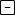.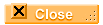Lesson 2 Answer 1 1.2.1 the letter FAnswer 2 1.2.2 x =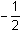Lesson 3 Answer 1 1.3.1 The other root is x = – 0.5 .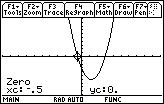Lesson 4 Answer 1 1.4.1 x = -0.5Self Test Answer 1 Functions should be described symbolically, graphically and numerically. Answer 2 The Home screen is the screen on the TI-89 where commands are entered and the results of the commands are shown. Answer 3 Enter the following commands: Define g(x)=2x^3–x^2–8x+4 Solve(g(x)=0,x)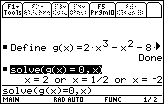The roots of g are x = 2, x =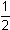, and x = –2. Answer 4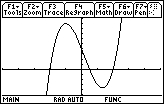Answer 5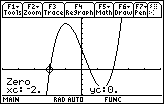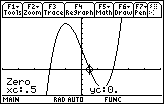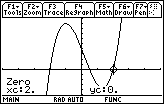Answer 6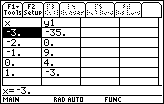Answer 7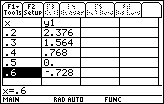©Copyright 2007 All rights reserved. | Trademarks | Privacy Policy | Link Policy